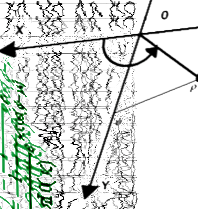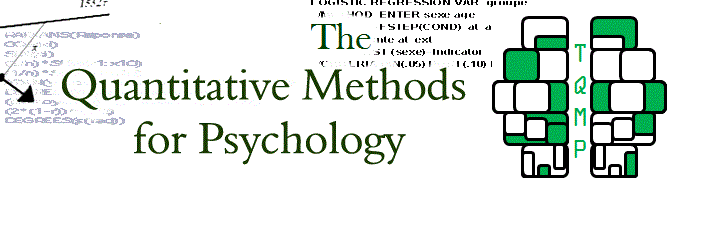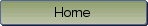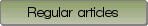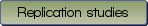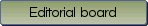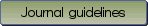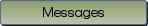Partial Least Squares tutorial for analyzing neuroimaging data

Bibliographic information: BibTEX format RIS format XML format APA style
Cited references information: BibTEX format APA style

Van Roon, Patricia , Zakizadeh, Jila , Chartier, Sylvain
200-215
Keywords: Partial least squares , PLS , regression , correlation
Tools: Mathematica
(no sample data)   (Appendix)

Partial least squares (PLS) has become a respected and meaningful soft modeling analysis technique that can be applied to very large datasets where the number of factors or variables is greater than the number of observations. Current biometric studies (e.g., eye movements, EKG, body movements, EEG) are often of this nature. PLS eliminates the multiple linear regression issues of over-fitting data by finding a few underlying or latent variables (factors) that account for most of the variation in the data. In real-world applications, where linear models do not always apply, PLS can model the non-linear relationship well. This tutorial introduces two PLS methods, PLS Correlation (PLSC) and PLS Regression (PLSR) and their applications in data analysis which are illustrated with neuroimaging examples. Both methods provide straightforward and comprehensible techniques for determining and modeling relationships between two multivariate data blocks by finding latent variables that best describes the relationships. In the examples, the PLSC will analyze the relationship between neuroimaging data such as Event-Related Potential (ERP) amplitude averages from different locations on the scalp with their corresponding behavioural data. Using the same data, the PLSR will be used to model the relationship between neuroimaging and behavioural data. This model will be able to predict future behaviour solely from available neuroimaging data. To find latent variables, Singular Value Decomposition (SVD) for PLSC and Non-linear Iterative PArtial Least Squares (NIPALS) for PLSR are implemented in this tutorial. SVD decomposes the large data block into three manageable matrices containing a diagonal set of singular values, as well as left and right singular vectors. For PLSR, NIPALS algorithms are used because it provides amore precise estimation of the latent variables. Mathematica notebooks are provided for each PLS method with clearly labeled sections and subsections. The notebook examples show the entire process and the results are reported in the Section 3 Examples.

Be informed of the upcoming issues with RSS feed:RSS